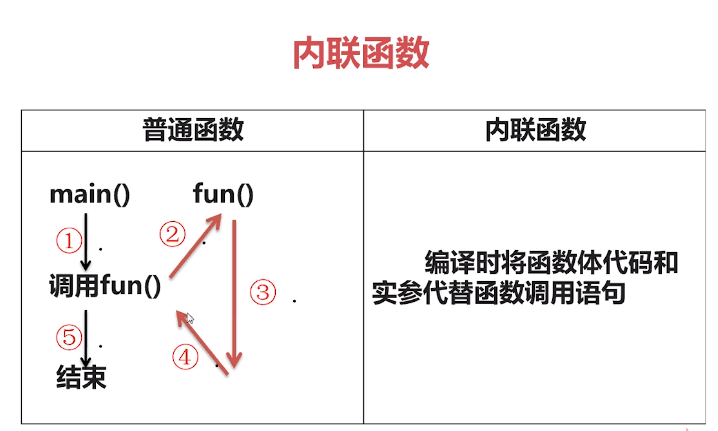# c++学习笔记 const 和 function

By 依韵 , From https://blog.cdswyda.com/post/the-const-and-function-of-cpp

## const

```// const.cpp: 定义控制台应用程序的入口点。
//

#include "stdafx.h"
#include <iostream>

using namespace std;

void output1(int a, int b);

void output2(const int &a, const int &b);

int main()
{
// 1 const base
int const a = 1;
const int b = 2;
// a = 11;  // 禁止修改
// b = 22;  // 禁止修改
// ===========================================

// 2 const pointer
int c = 3;          // 变量x
const int d = 4;
const int *p1 = &c; // 指针指向x 指针禁止修改 但指针指向可变
// *p1 = 33; // 禁止修改
p1 = &d; // 指针p1不可更改对应的值，但是指针指向可变
cout << "修改后*p1 " << *p1 << endl;

int *const p2 = &c;
*p2 = 33;
cout << "*p2修改c " << c << endl;
//p2 = &d; // 禁止修改

// 总结：
// const 修饰的是距离const最近的
// const int *p1 = &c;  此时 const 修饰 *p1 禁止指针修改值 即指针为只读指针 但是指针可以重新指向
// int *const p2 = &c;  此时 const 修饰 p2 禁止指针指向修改 指针本身可读可写

const int e = 5;
// int *p3 = &e;
// 上一行禁止 原因： e为常量，但是指针指向e，指针可读可写，存在矛盾风险，禁止

const int *p3 = &e;
// 上一行则允许，因为指针p3只可读 并不可写
cout << "指针p3 " << *p3 << endl;

// int *const p4 = &e;
// 上行依然禁止，原因同上，此时不可变的仅仅是指针p4的指向 并非指针p3本身

// 但是p3仍然存在一个风险 即p3指向可变，如：
p3 = &c;
cout << "*p3指针指向被修改 " << *p3 << endl;
// 因此可以同时是的使得指针和指向都不可变
const int *const p5 = &e;
// *p5 = 55； // 禁止
// p5 = &c; // 禁止

// ==============================

// 3 const reference
int m = 10;
int n = 100;
output1(m, n);

int x = 10;
int y = 100;
output2(x, y);

system("pause");
return 0;
}

void output1(int a, int b) {
// 此处意外地修改了传入的参数
a *= a;
b *= b;
cout << a << "," << b << endl;
}

void output2(const int &a, const int &b) {
// 这时候传入的参数是对实参的静态引用
// 以下的两行修改则会直接报错
// a *= a;
// b *= b;
cout << a << "," << b << endl;
}
```

## function

### 函数默认值

c++中函数支持默认值，但是有一个规则限制，即：若存在默认值，则有默认值的参数必须在最右端。

```void fun(int a, int b = 2, int c); // 错误,默认实参不在形参列表的结尾

// 正确的应为：
void fun(int a, int b = 2, int c = 3);
// 可以这么理解，如果给一个参数指定了默认值
// 则必须给这个参数之后的每个参数都加入默认值。
```

### 重载

1. 同一个作用域
2. 同名函数
3. 形参的个数不同或者某个参数的类型不同

### 内联函数• 内联编译是建议性质的，具体还是由编译器决定
• 只是用于逻辑简单的情景，不能包含 `for``while` 循环等复杂的结构。
• 递归函数无法使用内联方式
```// inline
inline double f1(double a, double b)
{
return a + b;
}
double f2(double a, double b)
{
return a + b;
}

int main () {
int i = 0;
const double pi = 3.141592652;
clock_t t1 = clock();
do {
f2(pi, pi);
} while (i++ < 100000000);
cout << "normal " << (clock() - t1) * 1.0 / CLOCKS_PER_SEC * 1000 << endl;

i = 0;
clock_t t2 = clock();
do {
f1(pi, pi);
} while (i++ < 100000000);
cout << "inline " << (clock() - t2) * 1.0 / CLOCKS_PER_SEC * 1000 << endl;
return 0;
}
```